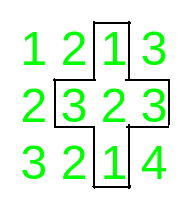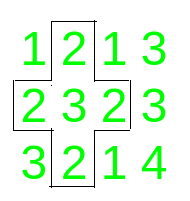# Count of palindromic plus paths in a given Matrix

• Last Updated : 15 Jun, 2021

Given an N x M matrix of integers, the task is to count the number of palindromic pluses in the array.

Palindromic plus is formed when a palindromic sub-row and palindromic sub-column cross each other at the middle element.

Examples:

Input: matrix = [[1, 2, 1], [2, 3, 2], [3, 2, 1]]
Output:
Explanation:
Palindromic row from (1, 0) – > (1, 2) and Palindromic column (0, 1) -> (2, 1) form a palindromic plus.
Input: matrix = [[1, 2, 1, 3], [2, 3, 2, 3], [3, 2, 1, 4]
Output:
Explanation:
The palindromic pluses in the given matrix are:Approach:
To solve the problem, follow the steps below:

• Traverse all the cells that can be the center of a palindromic plus, that is, all the cells apart from the ones belonging to the first and last row and columns.
• For all these cells (i, j), check if a[i][j – 1] is equal to a[i][j + 1] and a[i – 1][j] is equal to a[i + 1][j]. If both the conditions satisfies, then increase the count of palindromic pluses.
• Print the final count of palindromic pluses.

Below is the implementation of the above approach:

## C++

 `// C++ Program to count the number` `// of palindromic pluses in` `// a given matrix` `#include ` `using` `namespace` `std;`   `// Function to count and return` `// the number of palindromic pluses` `int` `countPalindromicPlus(` `    ``int` `n, ``int` `m,` `    ``vector >& a)` `{` `    ``int` `i, j, k;` `    ``int` `count = 0;`   `    ``// Traverse all the centers` `    ``for` `(i = 1; i < n - 1; i++) {` `        ``for` `(j = 1; j < m - 1; j++) {`   `            ``// Check for palindromic plus` `            ``// Check whether row and` `            ``// column are palindrome or not` `            ``if` `(a[i + 1][j] == a[i - 1][j]` `                ``&& a[i][j - 1] == a[i][j + 1])` `                ``++count;` `        ``}` `    ``}` `    ``// Return the answer` `    ``return` `count;` `}`   `// Driver code` `int` `main()` `{` `    ``int` `n = 4, m = 4;`   `    ``vector > a` `        ``= { { 1, 2, 1, 3 },` `            ``{ 2, 3, 2, 3 },` `            ``{ 3, 2, 1, 2 },` `            ``{ 2, 3, 2, 3 } };` `    ``cout << countPalindromicPlus(` `                ``n, m, a)` `         ``<< endl;`   `    ``return` `0;` `}`

## Java

 `// Java program to count the number` `// of palindromic pluses in` `// a given matrix` `class` `GFG{`   `// Function to count and return` `// the number of palindromic pluses` `static` `int` `countPalindromicPlus(``int` `n, ``int` `m,` `                                ``int` `[][]a)` `{` `    ``int` `i, j;` `    ``int` `count = ``0``;`   `    ``// Traverse all the centers` `    ``for``(i = ``1``; i < n - ``1``; i++)` `    ``{` `       ``for``(j = ``1``; j < m - ``1``; j++)` `       ``{` `          `  `          ``// Check for palindromic plus` `          ``// Check whether row and` `          ``// column are palindrome or not` `          ``if` `(a[i + ``1``][j] == a[i - ``1``][j] && ` `              ``a[i][j - ``1``] == a[i][j + ``1``])` `              ``++count;` `       ``}` `    ``}` `    `  `    ``// Return the answer` `    ``return` `count;` `}`   `// Driver code` `public` `static` `void` `main(String[] args)` `{` `    ``int` `n = ``4``, m = ``4``;` `    ``int` `[][]a = { { ``1``, ``2``, ``1``, ``3` `},` `                  ``{ ``2``, ``3``, ``2``, ``3` `},` `                  ``{ ``3``, ``2``, ``1``, ``2` `},` `                  ``{ ``2``, ``3``, ``2``, ``3` `} };` `                  `  `    ``System.out.print(` `           ``countPalindromicPlus(n, m, a) + ``"\n"``);` `}` `}`   `// This code is contributed by amal kumar choubey`

## Python3

 `# Python3 Program to count the number` `# of palindromic pluses in` `# a given matrix`   `# Function to count and return` `# the number of palindromic pluses` `def` `countPalindromicPlus(n, m, a):` `    ``i, j, k ``=` `0``, ``0``, ``0` `    ``count ``=` `0`   `    ``# Traverse all the centers` `    ``for` `i ``in` `range``(``1``, n ``-` `1``):` `        ``for` `j ``in` `range``(``1``, m ``-` `1``):`   `            ``# Check for palindromic plus` `            ``# Check whether row and` `            ``# column are palindrome or not` `            ``if` `(a[i ``+` `1``][j] ``=``=` `a[i ``-` `1``][j]` `                ``and` `a[i][j ``-` `1``] ``=``=` `a[i][j ``+` `1``]):` `                ``count ``+``=` `1` `                `  `    ``# Return the answer` `    ``return` `count`   `# Driver code` `if` `__name__ ``=``=` `'__main__'``:` `    ``n ``=` `4` `    ``m ``=` `4`   `    ``a ``=` `[[``1``, ``2``, ``1``, ``3` `],` `         ``[``2``, ``3``, ``2``, ``3` `],` `         ``[``3``, ``2``, ``1``, ``2` `],` `         ``[``2``, ``3``, ``2``, ``3` `]]` `    ``print``(countPalindromicPlus(n, m, a))`   `# This code is contributed by Mohit Kumar`

## C#

 `// C# program to count the number` `// of palindromic pluses in` `// a given matrix` `using` `System;` `class` `GFG{`   `// Function to count and return` `// the number of palindromic pluses` `static` `int` `countPalindromicPlus(``int` `n, ``int` `m,` `                                ``int` `[,]a)` `{` `    ``int` `i, j;` `    ``int` `count = 0;`   `    ``// Traverse all the centers` `    ``for``(i = 1; i < n - 1; i++)` `    ``{` `        ``for``(j = 1; j < m - 1; j++)` `        ``{` `            `  `            ``// Check for palindromic plus` `            ``// Check whether row and` `            ``// column are palindrome or not` `            ``if` `(a[i + 1, j] == a[i - 1, j] && ` `                ``a[i, j - 1] == a[i, j + 1])` `                ``++count;` `        ``}` `    ``}` `    `  `    ``// Return the answer` `    ``return` `count;` `}`   `// Driver code` `public` `static` `void` `Main()` `{` `    ``int` `n = 4, m = 4;` `    ``int` `[,]a = {{ 1, 2, 1, 3 },` `                ``{ 2, 3, 2, 3 },` `                ``{ 3, 2, 1, 2 },` `                ``{ 2, 3, 2, 3 }};` `                `  `    ``Console.Write(` `            ``countPalindromicPlus(n, m, a) + ``"\n"``);` `}` `}`   `// This code is contributed by Code_Mech`

## Javascript

 ``

Output:

`3`

Time Complexity: O(N2
Auxiliary Space: O(1)

My Personal Notes arrow_drop_up
Recommended Articles
Page :